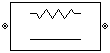# Series R

Model series resistor

• Library:
• RF Blockset / Equivalent Baseband / Series / Shunt RLC

•## Description

The Series R block models the series resistor described in the block dialog box, in terms of its frequency-dependent S-parameters.

The series R object is a two-port network, as shown in the following circuit diagram.## Parameters

expand all

### Main

Resistance value, specified as a scalar.

### Visualization

Source of frequency data, specified as `User-Specified`.

Frequencies, specified as vector in hertz..

Impedance to use when plotting small-signal parameters, specified as scalar in ohms.

Plot type, specified as `Composite data`, `X-Y plane`, ```Polar plane```, `Z Smith chart`, `Y Smith chart`, or ```ZY Smith chart```. For more information see, Create Plots.

First parameter on Y-axis, specified as `S11`, `S12`, `S21`, `S22`, `GroupDelay`, `OIP3`, `NF`, `NFactor`, or `NTemp`.

Second parameter on Y-axis (optional), specified as `S11`, `S12`, `S21`, `S22`, `GroupDelay`, `OIP3`, `NF`, `NFactor`, or `NTemp`.

Plot format, specified as `Magnitude (decibels)`, `Angle(degrees)`, `Real`, or `Imaginary`.

Plot format, specified as `Magnitude (decibels)`, `Angle(degrees)`, `Real`, or `Imaginary`.

Y-axis scale, specified as `Linear` or `Logarithmic`.

X-axis scale, specified as `Linear` or `Logarithmic`.

Plot specified data using plot button.